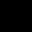# The number of prime factors in the expression (6)10 x (7)17 x (11)27 is

## One Answer on this Question

1.Rahul Kumar Tiwary says:

610 × 717 × 1127
= (2 × 3)10 × 717 × 1127
= 210 × 310 × 717 × 1127
Number of prime factors in the given expression
= (10 + 10 + 17 + 27)
= 64

Scroll to top